Dipping a long box multiple times into a shorter box

mbroowil

New member
A short box is full of liquid cleaning solution. A longer box needs to be cleaned with the liquid solution but does not fit entirely inside the short box.

1) Can the box be entirely cleaned?
2) If not, how much area will be missed?
3) If so, how many dips are required?
4) If just 2 dips are required, can they be done without having to turn the longer box upside down?

I currently just use a CAD software to find the answer through trial-and-error but academically I'd like to know a concise mathematical solution for it.

First, let's assume we can ignore depth and just focus on length and width.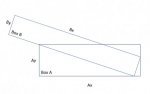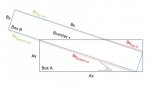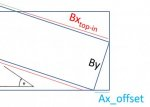I'm not sure how to calculate Ax_offset without trial-and-error. The angle theta is based on the offset, but the offset is based on the angle.

Then it seems like a long route to calculate the remaining items to start answering questions.

If Bx_top_in is greater than half of Bx, I think it can be dipped in 2 dips without having to flip the box upside down.

If Bcenter falls inside of the small box but Bx_top_in is less than half of Bx, then I think it can be dipped in 2 dips but the longer box must be flipped upside down on the 2nd dip.

I can't currently imagine a scenario where 4 dips would accomplish anything 2 dips (with a flip) wouldn't.

Can anyone help with some strategies for solving (long-hand... for fun) the original questions.

Mike

Dr.Peterson

Elite Member
To find Ax_offset, I would use the similar right triangles with legs along A_x. Similarity gives you a proportion, and the Pythagorean Theorem gives you another equation involving the same two variables (Ax_offset and the other leg of that right triangle). Eliminate the other variable, and solve for Ax_offset.

Is theta supposed to be the angle between box B and the horizontal? The figure doesn't show it as such.

mbroowil

New member
Thanks for the response!

Yes, theta was the angle between Box B's B_x_bottom and Box A's A_x_bottom.

Prior to solving, my knowns are A_x, A_y, B_x, and B_y. With that... I also can define all of the interior box angles as 90 degrees.

I'm still confused for solving for A_x_offset to start answering all of the other questions. I know B_y and A_y. Could you help me understand your suggestion?

I've labelled a few triangles that are created by different B_y's (B_y = 0, 0 < B_y < A_y, and B_y > A_y) in the diagrams below.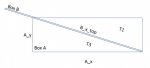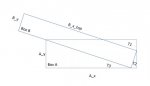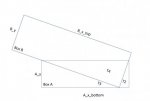T1 is the triangle formed above Box B.
T2 is the triangle formed by the horizontal offset.
T3 is the triangle formed beneath Box B.
T4 is the triangle formed in Box B.

Any help with understanding the similar triangles you suggested would be appreciated.

Thanks again,
Mike

Dr.Peterson

Elite Member
Thanks for the response!

Yes, theta was the angle between Box B's B_x_bottom and Box A's A_x_bottom.

Prior to solving, my knowns are A_x, A_y, B_x, and B_y. With that... I also can define all of the interior box angles as 90 degrees.

I'm still confused for solving for A_x_offset to start answering all of the other questions. I know B_y and A_y. Could you help me understand your suggestion?

I've labelled a few triangles that are created by different B_y's (B_y = 0, 0 < B_y < A_y, and B_y > A_y) in the diagrams below.

View attachment 11041

View attachment 11042

View attachment 11043

T1 is the triangle formed above Box B.
T2 is the triangle formed by the horizontal offset.
T3 is the triangle formed beneath Box B.
T4 is the triangle formed in Box B.

Any help with understanding the similar triangles you suggested would be appreciated.
I was referring to your triangles T2 and T3 (for the situation in your first two pictures above; the last is much easier).

Try writing the equations I suggested, and we can discuss it further.

mbroowil

New member
I don't understand fundamentally how we know T2 and T3 are similar triangles, but I'll do some googling on that to convince myself later.

Once they are identified as similar triangles, google tells me we can use the following scale factor:

SU / NQ = ST / NP = UT
or
SU / A_y* = B_y* / NP = UT / QP
or
A_x_T2 / A_y* = B_y* / NP = UT / (A_x* - A_x_T2)

*'s are known.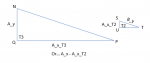T2 has been rotated for ease of visualization. Corners have been labeled arbitrarily for ease of communication.

So, knowing nothing but A_y, A_x, B_y, and B_x... and using the scale factor for similar triangles... do I have enough enough known to reduce and solve the simultaneous equations (another google search when I have some time)?

Thanks again,
Mike

Dr.Peterson

Elite Member
I don't understand fundamentally how we know T2 and T3 are similar triangles, but I'll do some googling on that to convince myself later.

Once they are identified as similar triangles, google tells me we can use the following scale factor:

SU / NQ = ST / NP = UT
or
SU / A_y* = B_y* / NP = UT / QP
or
A_x_T2 / A_y* = B_y* / NP = UT / (A_x* - A_x_T2)

*'s are known.
View attachment 11050

T2 has been rotated for ease of visualization. Corners have been labeled arbitrarily for ease of communication.

So, knowing nothing but A_y, A_x, B_y, and B_x... and using the scale factor for similar triangles... do I have enough enough known to reduce and solve the simultaneous equations (another google search when I have some time)?

Thanks again,
Mike
The reason you can know they are similar triangles is that your angles S and P add up to 90 degrees, so angles S and N are congruent.

Combing your equation A_x_T2 / A_y* = B_y* / NP and the Pythagorean theorem NP^2 = (A_x* - A_x_T2)^2 + A_y^2 constitute a system of two equations in two unknowns, which in principle could be solved.

Unfortunately, I get a 4th degree equation when I eliminate a variable, which is not very nice to solve. (I've seen a lot of problems similar to this one that work our similarly.) It may be that we can't find a formula, but would need numerical methods.

mbroowil

New member
Dr. Peterson, thank you so much for your continued help on this!

To circle back on my original thoughts (geometry pun intended)...

Right now I draw the two boxes in CAD and move/rotate/move/rotate until I can get the the correct angle (theta) & horizontal offset (A_x_offset) combination. It works, and it works quickly, and it works easily... but I thought it would be fun to solve for theta before opening a CAD application (and I thought it was embarrassing that it wasn't obvious to me how I would solve for this)...

But, if I understand correctly, it's not a VERY simple formulaic solution... but instead would use some numerical approximation and/or graphing? Which suddenly makes my move/rotate/move/rotate solution seem less embarrassing.

And from there.... I think all of the rest of my questions can be simplified to:

Rule 1) If B_x_top_in is greater than 0.5 * Bx then it can be cleaned in 2 dips without flipping the longer box upside down.
Rule 2) If "Rule 1" is not true and B_center is "inside" box A then the box can be cleaned in 2 dips but flipping the longer box upside down is required.
Rule 3) As long as B_y < A_y there is no need to dip something more than twice.

I'll think about more generic solutions for general solutions to dipping a box in a box... and maybe share an excel workbook or something.

Thanks again for all the help!

-Mike

Last edited:

Dr.Peterson

Elite Member
Dr. Peterson, thank you so much for your continued help on this!

To circle back on my original thoughts (geometry pun intended)...

Right now I draw the two boxes in CAD and move/rotate/move/rotate until I can get the the right angle (theta) & horizontal offset (A_x_offset) combination. It works, and it works quickly, and it works easily... but I thought it would be fun to solve for theta before opening a CAD application (and I thought it was embarrassing that it wasn't obvious to me how I would solve for this)...

But, if I understand correctly, it's not a VERY simple formulaic solution... but instead would use some numerical approximation and/or graphing? Which suddenly makes my move/rotate/move/rotate solution seem less embarrassing.

And from there.... I think all of the rest of my questions can be simplified to:

Rule 1) If B_x_top_in is greater than 0.5 * Bx then it can be cleaned in 2 dips without flipping the longer box upside down.
Rule 2) If "Rule 1" is not true and B_center is "inside" box A then the box can be cleaned in 2 dips but flipping the longer box upside down is required.
Rule 3) As long as B_y < A_y there is no need to dip something more than twice.

I'll think about more generic solutions for general solutions to dipping a box in a box... and maybe share an excel workbook or something.

Thanks again for all the help!

-Mike
Yes, quite likely the best way to solve it besides your CAD work would be to write an equation (perhaps my quartic, or even the system of equations from which I got it), and use something like a graphing calculator or solving capability in a spreadsheet to solve it numerically.